# Sensors and Transducers

## Sensors and Transducers:

The definition of Sensors and Transducers are follows,

The input quantity for most instrumentation systems is nonelectrical. In order to use electrical methods and techniques for measurement, the nonelectrical quantity is converted into a proportional electrical signal by a device called “transducer”.

Actually, electrical transducer consists of two parts which are very closely related to each other. These two parts are sensing or detecting element and transduction element. The sensing or detecting element is commonly known as sensor.

Let us discuss the operation and applications of different types of available Sensors and Transducers.

### Light Sensors:

The light dependent resistor (LDR) is used as a light sensor. It is made of cadmium sulphide or cadmium selenide whose resistance depends on amount of light present. The Fig. 1529 shows the light control relay circuit using a photoresistor.The resistance of photoresistor varies from about 15 MΩ when in the dark to about 15 KΩ when in a bright light. When resistance of photoresistor is high there is a sufficient bias voltage for darlington transistors making them to conduct. This energies the relay. On the other hand, when resistance of photoresistor is low (in bright light) there is no sufficient bias voltage for darlington transistors. As a result they do not conduct and relay is not energised. Like photoresistance there are many light sensing devices like photodiode and phototransistor.

In photodiodes the reverse leakage current increases linearly as the amount of light falling on it increases. The Fig. 15.30 shows the light sensor circuitry using photodiode. Here, the small leakage current is converted into proportional voltage.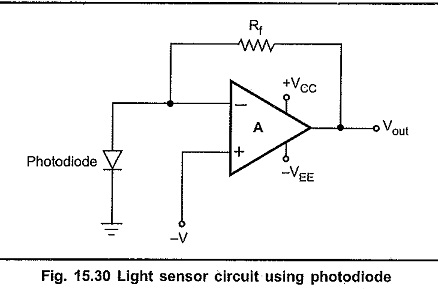The temperature sensors can be classified as semiconductor devices, thermocouples, RTDs and thermistors.

### Semiconductor Temperature Sensors:

The semiconductor temperature sensors are available in two types temperature sensitive voltage sources and temperature sensitive current sources. The Fig. 15.31 shows the temperature sensitive voltage source using LM35. The voltage output from this circuit increases by 10mV for each °c that its temperature is increased. If its output is connected to the negative reference voltage, – V, as shown in Fig. 15.31, the sensor will give a output for temperature range of – 55 to + 150°c.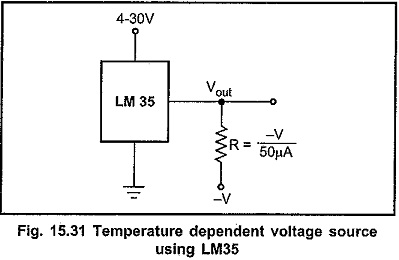Analog device AD590 is a temperature dependent current source. It produces a current of 1 µA/°K. This current can be converted into voltage by current-voltage converter using op-amp.

### Thermocouple:

A thermocouple consists of a pair of dissimilar petal wires joined together at one end, forming a hot junction and terminated at the other end known as reference or cold junction. When heat is applied to the hot junction, a temperature difference exists between the hot junction and the cold junction, causing generation of emf. This is illustrated in Fig. 15.32 (a). The magnitude of this emf depends on the material used for the wires and the temperature difference between the two junctions. The two dissimilar metals form an electric circuit, and a current flows as a result of the generated emf as shown in Fig. 15.32 (a).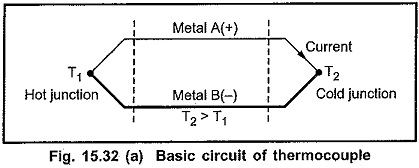When the cold junction is terminated by a meter or recording instrument, as shown in Fig. 15.32 (b), the meter indication is proportional to the thermoelectric effect, caused by constant potentials at the junctions, is known as the seeback effect.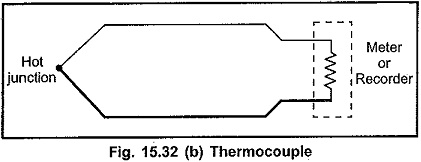#### Advantages of Thermocouple:

1. The thermocouple is rugged in construction.
2. It covers a wide temperature range, from —270°c to 2700°c.
3. Using extension leads and compensating cables, long transmission distances for temperature measurement are possible. This is most suitable for temperature measurement of industrial furnaces.
4. The thermocouple is comparatively cheaper in cost.
5. The calibration can be easily checked.
6. The thermocouple offers good reproducibility.
7. Speed of response is high.
8. Measurement accuracy is quite satisfactory.

#### Limitations of Thermocouple:

1. For accurate temperature measurements, cold junction compensation is
2. The emf induced verus temperature characteristics is somewhat nonlinear.
3. Stray voltage pickup is possible.
4. In many applications, amplification of signal is required.

### Resistance Temperature Detectors [RTD]:

Generally, electrical resistance of any metallic conductor varies according to temperature changes. The sensor for measurement of temperature by utilizing this phenomenon is called “Resistance Thermometer”. It is a basic element of resistance temperature detector, RTD.

Measurement of change of resistance of RTD due to temperature changes is measured by Wheatstone bridge. The Fig. 15.33 shows the necessary circuit connections.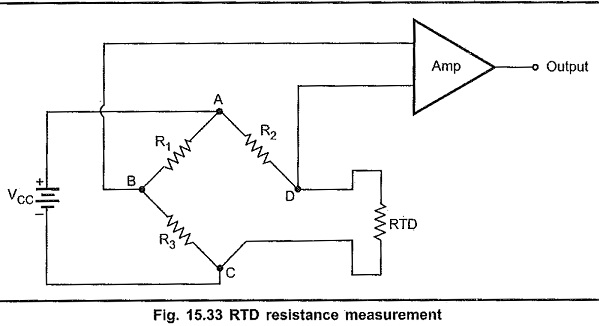At 0°C, the resistance of RTD is usually 100 Ω. By choosing R3 = 100 Ω and R1= R2, the bridge is balanced at O°C. Therefore, at 0°C voltage across B and D is zero and hence the output voltage is zero. Any change in the RTD resistance due to change in temperature unbalances the bridge circuit resulting voltage across B and D terminal. This voltage is proportional to the change in the resistance and hence to the change in the temperature.

The circuit just discussed is not suitable for high precision measurement because it is susceptible to lead resistance and produce error. For precision measurements 3-wire and 4-wire connection methods are used.

#### Advantages of RTD:

1. High accuracy
2. They can be calibrated to detect the actual temperatures to within ± 0.25°C up to 120°C and ± 0.5°C from 120°C to 550°C. Therefore they have wide temperature range.
3. Does not require temperature compensation.
4. Designed for fast response.
5. Excellent stability and reproducibility.
6. Good sensitivity.

#### Disadvantages of RTD:

1. Requires bridge circuit and external power source for measurement.
2. Chances of self heating due to current through RTD and thus the change in resistance
3. Large size as compared to thermocouples
4. High cost

### Thermistors:

Thermistor is a contraction of a term ‘thermal-resistors‘. Thermistors are semiconductor device which behave as thermal resistors having negative temperature coefficient [NTC]; i.e. their resistance decreases as temperature increases.

Thermistors are composed of a sintered mixture of metallic oxides, such as manganese, nickel, cobalt, copper, iron, and uranium. Their resistances at ambient temperature may range from 100 Ω to 100 kΩ.

#### Advantages of Thermistor:

1. Small size and low cost.
2. Comparatively large change in resistance for a given change in temperature
3. -Fast response over a narrow temperature range.

#### Limitations of Thermistor:

1. The resistance versus temperature characteristic is highly non-linear.
2. Not suitable over a wide temperature range.
3. Because of high resistance of thermistor, shielded cables have to be used to minimize interference.

### Pressure Sensors:

Usually, strain guages or linear variable difference transformers (LVDTs) are used to convert pressure to a proportional electrical signal.

### Strain Guages:

A strain guage is a small resistor whose value changes when its length is changed. It may be made of thin wire, thin foil, or semiconductor material. The desirable characteristics of the strain gauge are gauge sensitivity, range of measurement, accuracy, frequency response, and the ambient environmental conditions it can withstand.

Sensitivity is defined as the smallest value of strain that can be measured. The maximum strain measurable and the accuracy achievable depend upon the type of gauges used and the method of gauging used.

The change in resistance of strain guage can be converted into voltage change by bridge circuit followed by an op-amp. The Fig. 15.34 shows the measurement of pressure using strain guage.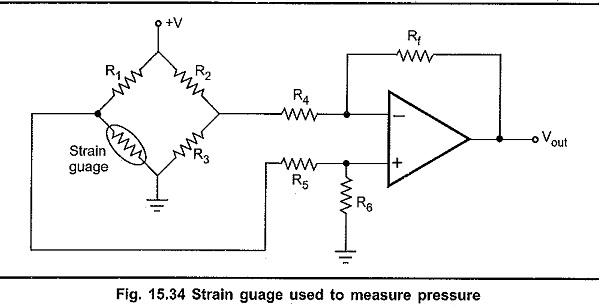The change in resistance due to change in pressure applied to strain guage unbalances the bridge and generates voltage different at the input of operational amplifier. This voltage difference is amplified using op-amp: The resulted output voltage is thus proportional to the change in strain guage resistance, i.e. change in applied pressure. The strain guage resistance also changes due to change in temperature. To get accurate reading it is necessary to compensate this change. This achieved by using two strain guages mounted at, right angles to each other and connected in two parallel arms of the bridge. Therefore, any change in the resistances of strain guages due to temperature have no effect on the differential output of the bridge.

### Linear Variable Differential Transformers (LVDT):

LVDT is an another type of transducer often used to measure force, pressure or position. The Fig. 15.35 shows the basic structure of LVDT. As illustrated in the Fig. 15.35, the linear variable differential transformer consists of a single primary winding P1 and two secondary windings S1 and S2 Wound on a hollow cylindrical former. The secondaries have an equal number of turns but they are connected in series opposition so that the emfs induced in the coils oppose each other. The primary winding is connected to an ac source, whose frequency may range from 50 Hz to 20 kHz. A movable soft iron core slides inside the hollow former The position of the movable core determines the flux linkage between the ac excited primary winding and each of the two secondary windings. The core made up, of nickel-iron alloy is slotted longitudinally to reduce eddy current losses. The displacement to be measured is applied to an arm attached to the core. With the core in the center, or reference, position, the induced emfs in the secondaries are equal, and since they oppose each other, the output voltage will be zero volt.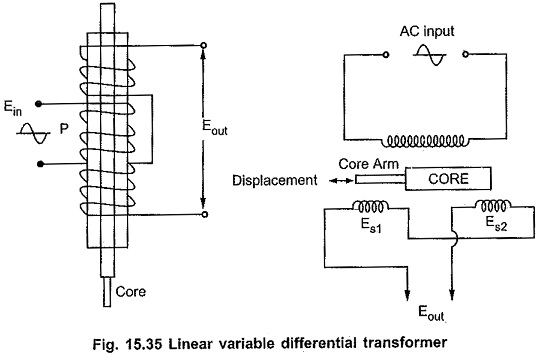When an externally applied force moves the core to the left-hand position, more magnetic flux links the left-hand coil than the right-hand coil. The emf induced in the left-hand coil, Es1, is therefore larger than the induced emf of the right-hand coil, Es2.

The magnitude of the output voltage is then equal to the difference between the two secondary voltages and it is in phase with the voltage of the left-hand coil.

Similarly, when the core is forced to move to the right, more flux links the right-hand coil than the left-hand coil and the resulting output voltage, which is the difference between Es2 and Es1, is now in phase with the emf of the right-hand coil.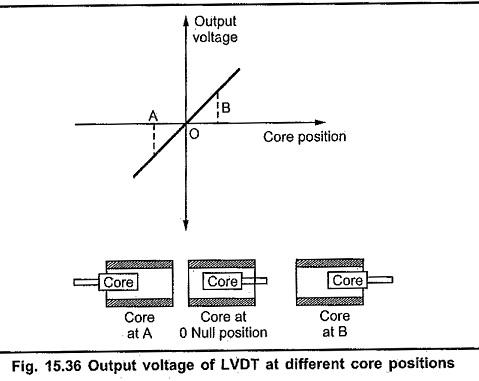Thus the LVDT output voltage is a function of the core position. The amount of a voltage change in either secondary winding is proportional to the amount of movement of the core. By noting which output is increasing or decreasing, the direction of motion can be determined. The output ac voltage inverts in phase as the core passes through

the central null position. Further as the core moves from the center, the greater is the difference in value between Es1 and Es2 and consequently the greater the output voltage. Therefore the amplitude of the output voltage is a function of the distance the core moves, while the polarity or phase indicates the direction of the motion.

The amount of output voltage of an LVDT is a linear function of the core displacement within a limited range of motion.

#### Advantages of LVDT:

1. Linearity : The output voltage of LVDT is almost linear for displacement upto
2. Infinite Resolution : The change in output voltage is continuous, stepless. The effective resolution depends more on the equipment used for the measurement rather than on the LVDT.
3. High Output : LVDT gives reasonably high output, and hence requires less amplification afterwards.
4. High Sensitivity : LVDT has high sensitivity of about 300 mV/mm; i.e., 1 mm displacement of the core produces a output voltage of 300 mV.
5. Ruggedness : LVDT is mechanically rugged and can withstand mechanical shock and vibrations.
6. Less Friction : Since there are no sliding contacts, the friction is very less.
7. Low Hysteresis : LVDT has a low hysteresis, hence its repeatability is extremely good under all conditions.
8. Low Power Consumption : Most LVDTs consume less than 1 W of power.
9. The LVDT transducers are small, simple, and light in weight. They are stable and easy to align and maintain.

#### Disadvantages of LVDT :

1. Comparatively large displacements are necessary for appreciable differential output.
2. They are sensitive to stray magnetic fields. However, his interference can be reduced by shielding.
3. The dynamic response is limited by the mass of the core.
4. Temperature affects the transducer.

#### Applications of LVDT :

• The LVDT can be used in all applications where displacement ranging from fractions of a few mm to a few cm have to be measured.
• Acting as a secondary transducer, LVDT can be used as a device to measure force, weight, and pressure etc. The force or pressure to be measured is first converted into a displacement using primary transducers. Then this displacement is applied to an LVDT, that acts as a secondary transducer, and converts the displacement into proportional output voltage. In these applications the high sensitivity of LVDT is a major attraction.

Uptil now we have seen various types of Sensors and Transducers. They convert physical, parameters into their electrical equivalent current or voltage. Once this conversion is over, we have to convert these analog electrical parameters into their digital equivalents. This can be done using A/D converters.